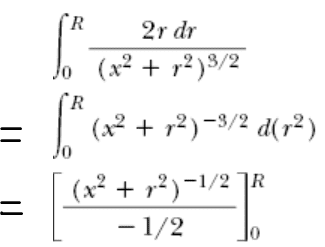# Solving integralHow does this work? I can only think that this is some sort of u-substitution of r^2 for r but then why don't the interval values change to 0 and R^2? Thanks!

When you do a u substitution the upper and lower bounds are only changed temporarily. Once you put back the original terms in place of u, the upper and lower bound go back to what they were in the first place, and then you do the final calculation of the definite integral. Since they didn't actually change to a new variable here, they never had to change the bounds.
Slight detail.

Also, don't let little r get confused with capital R. R is just some number.

SammyS
Staff Emeritus
Homework Helper
Gold Member
It's like a u substitution, but each step is in terms of r.

I suppose the proper thing to do would have been to write the second line as in one of the following forms:
$\displaystyle \int_0^{r=R}\left(x^2+r^2\right)^{-3/2}\,d(r^2)$

$\displaystyle \int_0^{\sqrt{R}}\left(x^2+r^2\right)^{-3/2}\,d(r^2)\,,$ since r2 is the "variable" of integration.​

But it's not unusual to see it expressed the way you posted it.

I always just change my upper and lower bounds to "a" and "b" until I'm back in the original terms. Too lazy to determine new bounds just to toss them out again, but careful enough not to lose points on an exam!

SammyS
Staff Emeritus
Homework Helper
Gold Member
I always just change my upper and lower bounds to "a" and "b" until I'm back in the original terms. Too lazy to determine new bounds just to toss them out again, but careful enough not to lose points on an exam!

You can usually work out the indefinite integral using whatever methods, writing the final result in terms of the original variable, of course, then put in the bounds for the definite integral.

You could also use a trigonometric function substitution, the denominator is a dead give away.

opposite = r
hypotenuse = sqrt(r^2 + x^2)

hypotenuse/opposite = csc(theta) = sqrt(r^2 + x^2)/r
csc(theta)^3 = (r^2 + x^2)^(3/2)/r^3

then

2 integral [0,R] r/(x^2+r^2)^(3/2) dr = 2 integral [0, R] dr/(r^3*csc(theta)^3)
I'll let you figure out were to go from there ^_^

HallsofIvy